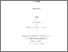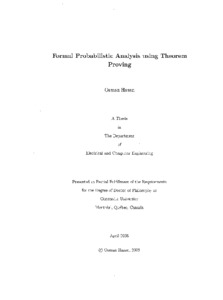Title:

# Formal probabilistic analysis using theorem proving

Hasan, Osman (2008) Formal probabilistic analysis using theorem proving. PhD thesis, Concordia University.Preview
Text (application/pdf)
NR37753.pdf - Accepted Version
6MB

## Abstract

Probabilistic analysis is a tool of fundamental importance to virtually all scientists and engineers as they often have to deal with systems that exhibit random or unpredictable elements. Traditionally, computer simulation techniques are used to perform probabilistic analysis. However, they provide less accurate results and cannot handle large-scale problems due to their enormous computer processing time requirements. To overcome these limitations, this thesis proposes to perform probabilistic analysis by formally specifying the behavior of random systems in higher-order logic and use these models for verifying the intended probabilistic and statistical properties in a computer based theorem prover. The analysis carried out in this way is free from any approximation or precision issues due to the mathematical nature of the models and the inherent soundness of the theorem proving approach. The thesis mainly targets the two most essential components for this task, i.e., the higher-order-logic formalization of random variables and the ability to formally verify the probabilistic and statistical properties of these random variables within a theorem prover. We present a framework that can be used to formalize and verify any continuous random variable for which the inverse of the cumulative distribution function can be expressed in a closed mathematical form. Similarly, we provide a formalization infrastructure that allows us to formally reason about statistical properties, such as mean, variance and tail distribution bounds, for discrete random variables. In order to in illustrate the practical effectiveness of the proposed approach, we consider the probabilistic analysis of three examples: the Coupon Collector's problem, the roundoff error in a digital processor and the Stop-and-Wait protocol. All the above mentioned work is conducted using the HOL theorem prover.

Divisions: Concordia University > Gina Cody School of Engineering and Computer Science > Electrical and Computer Engineering Thesis (PhD) Hasan, Osman xiv, 175 leaves : ill. ; 29 cm. Concordia University Ph. D. Electrical and Computer Engineering 2008 Tahar, Sofiène LE 3 C66E44P 2008 H37 975852 Concordia University Library 22 Jan 2013 16:16 13 Jul 2020 20:08 https://concordiauniversity.on.worldcat....
All items in Spectrum are protected by copyright, with all rights reserved. The use of items is governed by Spectrum's terms of access.

Repository Staff Only: item control pageResearch related to the current document (at the CORE website)
Back to top